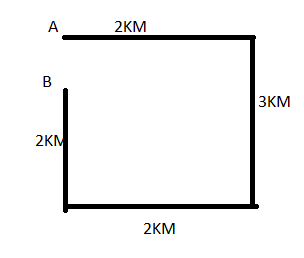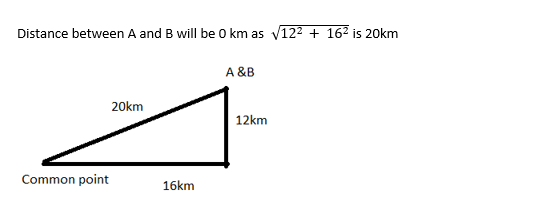# Wipro Logical Ability Previous Year Questions

28 January 2023

Wipro Logical Ability Previous Year Questions

Wipro Logical Ability Questions

Q1) In the following questions, a pair of numbers that have a certain relationship is given. One of the pairs given in four options made (1) to (4) bears the same relationship, find that pair.

36:1290

A. 20: 580

B. 25: 120

C. 25: 620

D. 18: 645

Explanation: 36: 1290

36*36 – 6 = 1290

Similarly

25*25 -5 = 620 hence option C

Q2) In the following questions, the alphabets bear a Random Sequence Numbering. Find the Numerals that would be represented by the following words.

If STREAM is written as 23 -24 -22 -9 -5 -17, how would you write BROOK?

A. 8 - 12 - 7 - 7 - 9

B. 6 - 22 - 19 - 19 - 15

C. 6 - 22 - 17 - 17- 7

D. 22 - 6 -  17 - 7 - 12

Explanation:

S   T     R    E  A  M

23 -24 -22 -9 -5 -17

As per alphabet number S is 19+4 = 23

Similarly, T -20+4 = 24

R – 18+4 =22

E – 5+4 =9

A -1+4 = 5

M – 13+4 = 17

Similarly for BROOK will be coded as 6 - 22 - 19 - 19 - 15

Q3) If the numbers of the clock are substituted by letters starting from 3 in an anticlockwise direction. 3 is substituted by C, 2 by E,1 by H, 12 by L and so on. Therefore by which letter will 10 be substituted?

A. U

B. W

C. X

D. V

Explanation: 3 in an anticlockwise direction.

3 is substituted by C, 2 by E after C, D will be skipped and E will be 2, after E F&G will be skipped similarly H will be 1 by skipping 3letters (I,J,K) , 12 by L and 11 by Q and 10 by W. Therefore, by which letter will 10 be substituted is W

Q4) In each of the following questions, the terms follow a definite pattern.

However, one term is wrong. Find the wrong term out of given options:

3,2, 8, 9, 13, 22, 18, 32, 23, 42

A. 8

B. 3

C. 22

D. 9

Explanation: The given sequence is a combination of two series:

I. 3, 8, 13, 18, 23 and II. 2, 9, 22, 32, 42

The pattern in I is + 5, and the pattern in II is + 10. So, in II, 9 is wrong and must be replaced by (2 + 10) i.e. 12.

Q5) The terms given in the following questions follow a definite pattern and thus make a series. Find the missing number from the series out of the given options:

20,19, 17, (--------)

A. 12

B. 13

C.15

D. 14

Explanation: 20 -1 = 19

19 -2 =17

17 – 3 = 14, hence option D

Q6) Five cars are parked in a row facing Eastward. E is parked to the left of A, B and C, B, C and A are parked to the left of D. C is parked between A and B. If B is parked fourth from the left, how far is A parked from the right?

A. Fourth

B. Third

C. Second

D. First

Explanation: E is parked to the left of A,B and C,

Current Order: E ABC

B,C A are parked to the left of D

Current Order: E ABC D

C is parked between A and B.

Current Order: E A/B C B/A D

B is parked fourth from the left.  Hence,

Current Order: E A C B D

A parked fourth from the right

Q7) From the given choices select the odd one out.

B. QTX

C. HKR

D. STE

Explanation: ADP -- 1,4,16 {1+4+16= 21}

QTX-- 17,20,24 {17+20+24= 61}

HKR-- 8,11,18 {8+11+18=37}

STE-- 19,20,5 {19+20+5 = 44}

except "STE”, other words produce odd results

Q8) From the given choices select the odd one out.

B. HKN

C. PSW

D. MPS

Explanation: A +3 – D+3 -G

H+3 – K+3 – N

P+3- S+4 – W

M+3 – P +3 – S

Only option C doesn’t follow the logic of +3, +3

Q9) The question consists of a problem question followed by two statements I and II. Find out if the information given in the statement(s) is sufficient in finding the solution to the problem.

Problem question: How much time would a machine take to put caps on 300 bottles?

Statements:

I. It takes 8 hours to put caps on 300 bottles manually.

II. It takes 2 minutes lesser to put cap on a bottle using machine than putting it manually.

A. Statement I alone is sufficient in answering the problem question

B. Statement II alone is sufficient in answering the problem question

C. Either of the statements taken individually is sufficient in answering the problem question

D. Both statements put together are sufficient in answering the problem question

E. Both the statements even put together are not sufficient in answering the problem question

Explanation: We have to find the time taken by a machine to put caps on 300 bottles.

The first statement gives the time taken to put caps on 300 bottles manually, but we have to find for a machine.

The second statement has a condition that states the time taken by a machine (a comparison of time taken).

So, we assume that we have to take both the 1st and the 2nd statement to find the required time taken by a machine.

Let's find the required time and see if our assumption is right or not.

It takes 8/300 hours = 480/300 minutes to cap a bottle, manually.

Then it will take (480/300 - 2) minutes = (480 - 600)/300 minutes = - 120/300 minutes to cap a bottle, using a machine.

Thus, it will take - 120/300 × 300 minutes = - 120 minutes = - 2 hours to cap 300 bottles.

Since time cannot be negative, then we can conclude that our assumption to determine time was wrong.

We understand that both the statements aren't sufficient to find the required time.

Q10) From the given choices select the odd one out.

A. AFB

B. MRN

C. KPL

D. RXS

Explanation: AFB --> 1, 6, 2 ==> {6-1=5, 6-2=4}

MRN --> 13, 18, 14 ==> {18-13=5, 18-14=4}

KPL --> 11, 16. 12 ==> {16-11=5, 16-12=4}

RXS --> 18, 24, 19 ==> {24-18=6, 24-19=5}

difference b/w 1st nd 2nd letter is 5 in all d words except "RXS"

Q11) Find the next number in the series.

1,4,18,48,....

A. 120

B. 64

C. 100

D. 96

Explanation: 1×1×1=1,

1×2×2=4,

2×3×3=18,

3×4×4=48,

4×5×5=100.

Q12) A man moves 2 kms towards east, then 3 kms towards South and again 2 kms towards west and then he goes 2 kms towards the initial point from where he started. In which direction is he from his initial position?

A. East

B. South

C. West

D. North

Explanation: He is in south direction with respective to the starting point.Q13) Two friends A and B start walking from a common point. A goes 20 kms towards north-east whereas B goes 16 kms towards east and then 12 kms towards north. How far are A and B from each other?

A. 14 kms

B. They are at the same place at the finishing point

C. 15 kms

D. Data is insufficient

Explanation:Q14) P is the father of R but is not his son. M is the spouse of R. How is M related to P?

A. Son-in-law

B. Wife

C. Daughter-in-law

D. Uncle

Explanation: P is the father of R.

R is not his son. It means R is not a boy, but a girl. So R is his daughter.

M is the spouse of R; which means M is married to R. So M is a man and husband of R.

M being related to P. M has married the daughter of P. It means that M is the son-in-law of P.

Thus, the answer is: M is related to P as his son-in-law.

Q15) Find the missing term in the series.

2,3,7,8,13,14……

A. 24

B. 21

C. 18

D. 20

Explanation: 2+1 = 3

3+4 = 7

7+1= 8

8+5 = 13

13+1 = 14

14+6 = 20

##